Factors What are factors Factors What are the

• Slides: 16Factors What are factors? ?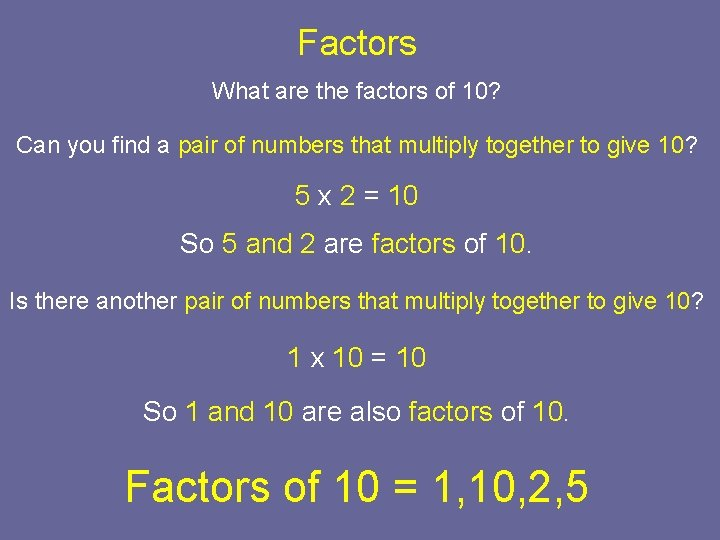Factors What are the factors of 10? Can you find a pair of numbers that multiply together to give 10? 5 x 2 = 10 So 5 and 2 are factors of 10. Is there another pair of numbers that multiply together to give 10? 1 x 10 = 10 So 1 and 10 are also factors of 10. Factors of 10 = 1, 10, 2, 5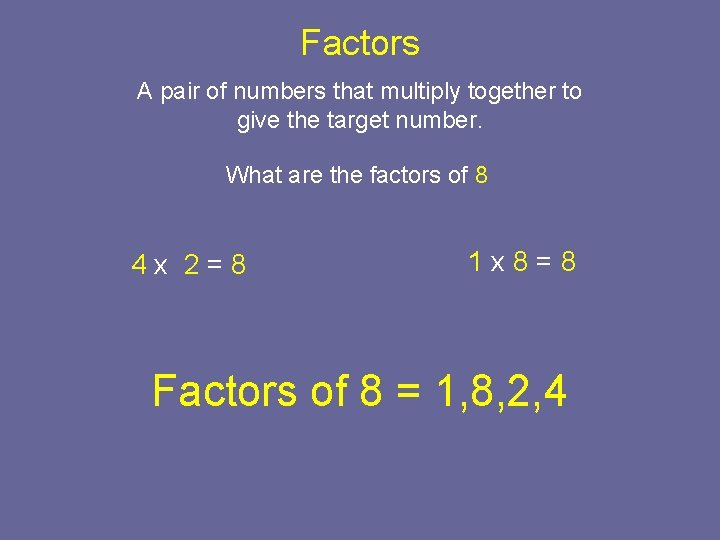Factors A pair of numbers that multiply together to give the target number. What are the factors of 8 4 x 2=8 1 x 8=8 Factors of 8 = 1, 8, 2, 4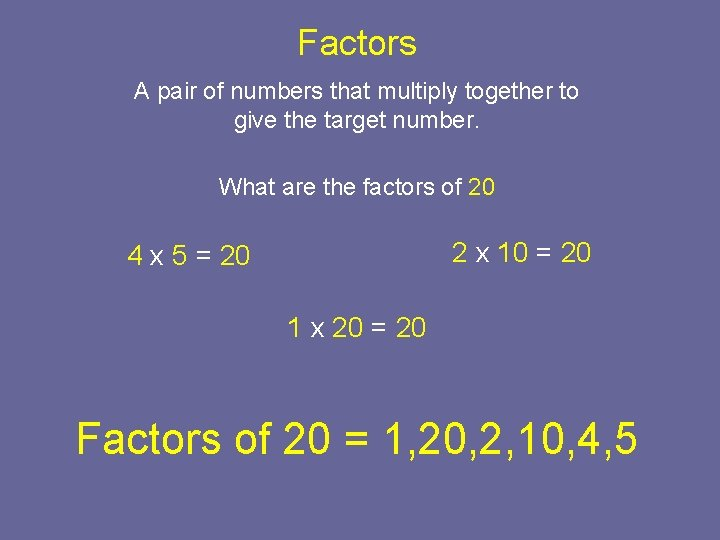Factors A pair of numbers that multiply together to give the target number. What are the factors of 20 2 x 10 = 20 4 x 5 = 20 1 x 20 = 20 Factors of 20 = 1, 20, 2, 10, 4, 5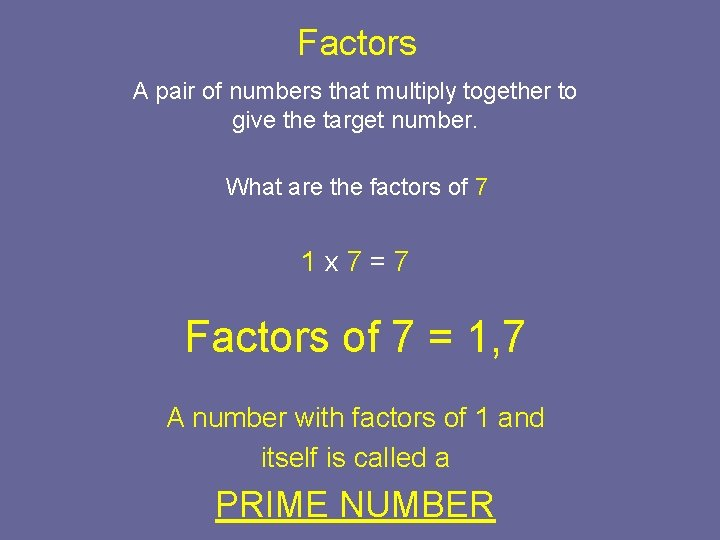Factors A pair of numbers that multiply together to give the target number. What are the factors of 7 1 x 7=7 Factors of 7 = 1, 7 A number with factors of 1 and itself is called a PRIME NUMBER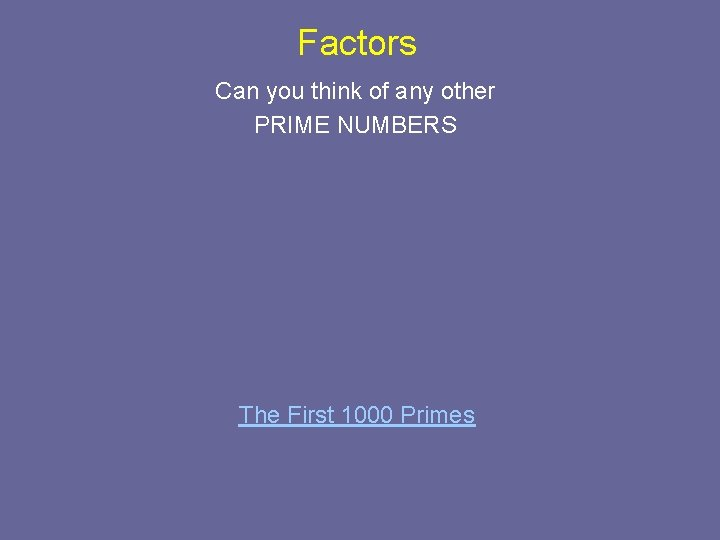Factors Can you think of any other PRIME NUMBERS The First 1000 Primes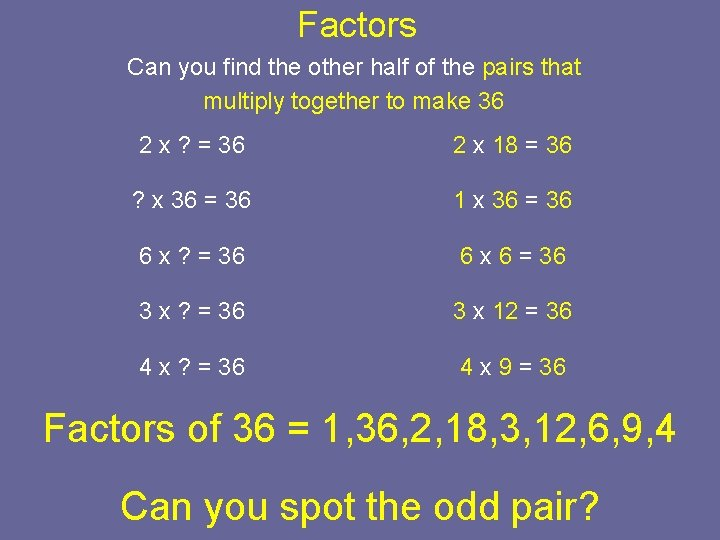Factors Can you find the other half of the pairs that multiply together to make 36 2 x ? = 36 2 x 18 = 36 ? x 36 = 36 1 x 36 = 36 6 x ? = 36 6 x 6 = 36 3 x ? = 36 3 x 12 = 36 4 x ? = 36 4 x 9 = 36 Factors of 36 = 1, 36, 2, 18, 3, 12, 6, 9, 4 Can you spot the odd pair?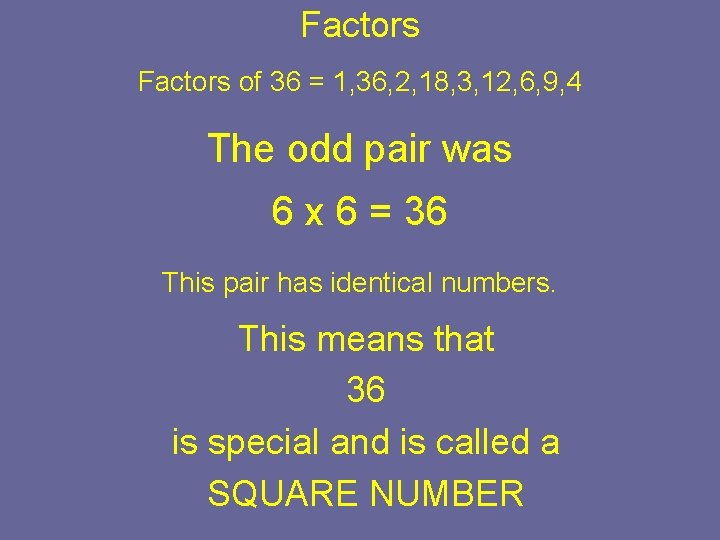Factors of 36 = 1, 36, 2, 18, 3, 12, 6, 9, 4 The odd pair was 6 x 6 = 36 This pair has identical numbers. This means that 36 is special and is called a SQUARE NUMBERSquare Numbers Can we find some more Square Numbers? ?We can use this idea of factors to help us with Multiplication. 4 x 2 x 3 0 r 3 x 2 x 4 0 r 4 x 3 x 2 Are there any other ways of doing this? Will we get the same answer every time?We can use this idea of factors to help us with Multiplication. 8 x 4 x 3 4 x 2 x 2 x 2 Which could be x 3 4 x 3 x 2 x 2 x 2 Which is 12 double x 10 2 x 20 4 x 40 2 20 4 2 40 8 2 80 8 16We can use this idea of factors to help us with Multiplication. 12 x 4 x 3 6 x 2 x 2 x 2 3 x 2 Which could be x 3 x 2 3 x 3 x 2 x 2 Which is 9 double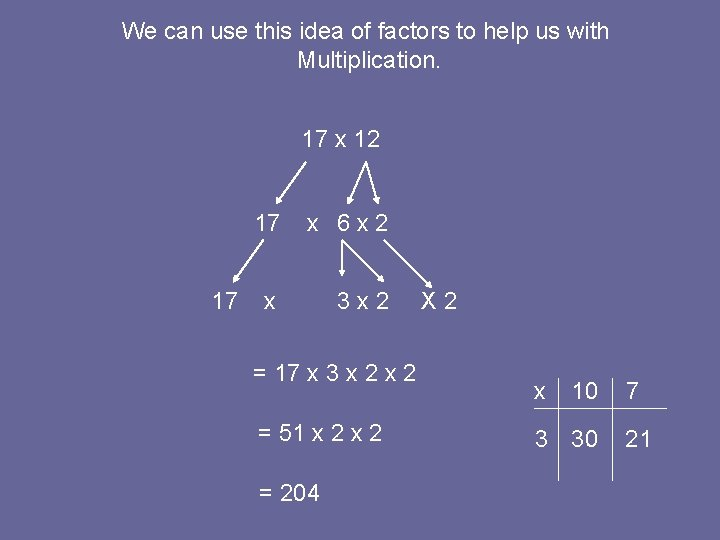We can use this idea of factors to help us with Multiplication. 17 x 12 17 17 x 6 x 2 x 3 x 2 = 17 x 3 x 2 = 51 x 2 = 204 X 2 x 10 3 30 7 21Another way of showing this is to record the factors horizontally. 26 x 6 = (13 x 2) x (3 x 2) = 13 x 2 x 2 = 39 x 2 x 2 = 78 x 2 = 156 x 10 3 3 30 9 x 9 30 2 60 18 x 8 70 2 140 16